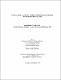## A primal-dual algorithm for the maximum charge problem with capacity constraints2010
##### Authors
Bhattacharjee, Sangita
University of Lethbridge. Faculty of Arts and Science
##### Publisher
Lethbridge, Alta. : University of Lethbridge, Dept. of Mathematics and Computer Science, 2010
##### Abstract
In this thesis, we study a variant of the maximum cardinality matching problem known as the maximum charge problem. Given a graph with arbitrary positive integer capacities assigned on every vertex and every edge, the goal is to maximize the assignment of positive feasible charges on the edges obeying the capacity constraints, so as to maximize the total sum of the charges. We use the primal-dual approach. We propose a combinatorial algorithm for solving the dual of the restricted primal and show that the primal-dual algorithm runs in a polynomial time.
##### Description
ix, 96 leaves : ill. ; 29 cm
##### Keywords
Matching theory , Combinatorial optimization , Graph theory , Dissertations, Academic